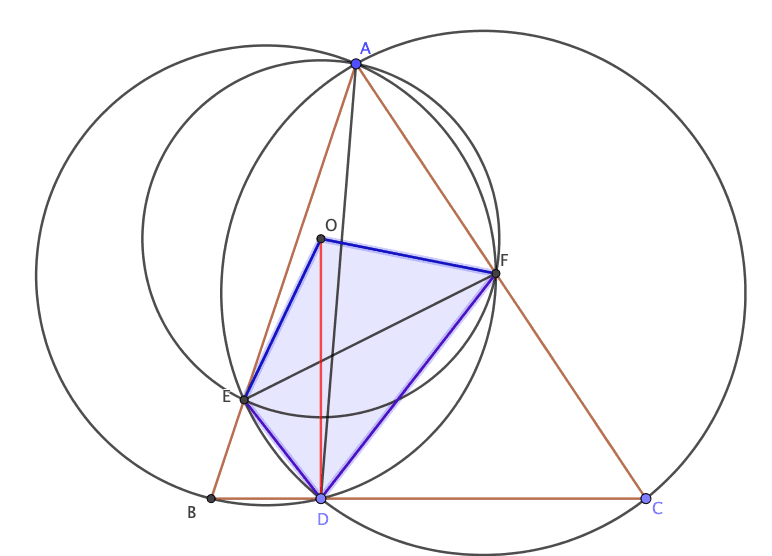How Cheenta works to ensure student success?
Explore the Back-Story

# RMO 2018 Tamil Nadu Problem 1 - angle bisectorRMO 2018 Tamil Nadu Problem 1 is from Geometry. We present sequential hints for this problem. Do not read all hints at one go. Try it yourself.

# Problem

Let ABC be an acute-angled triangle and let D be an interior point of the line segment BC. Let the circumcircle of triangle ACD intersect AB at E (E between A and B) and let the circumcircle of triangle ABD intersect AC at F (F between A and C). Let O be the circumcenter triangle AEF. Prove that OD bisects ( \angle EDF )

## Key ideas you will need to solve this problem

• Opposite angles of a cyclic quadrilateral adds up to $180^o$
• Angle at the center is twice the angle at the circumference.
• Angles subtended by the same arc at circumference are equal.

Also see

## Hint 1: Draw a picture## Hint 2: A construction would be nice

Join OE and OF. What kind of quadrilateral is OEDF? (Pause here. Try it yourself.)## Hint 3: Some angle chasing

Note that $EDCA$  is a cyclic quadrilateral (all of its four vertices are on a circle). Hence $\angle ODB = \angle EAC = \angle A$ .

Similarly $AFDB$ is cyclic. Hence $\angle CDF = \angle FAB = \angle A$

This implies $\angle EDF = 180^o - 2 \angle A$. Since O is the center of $\Delta AEF$, $\angle EOF = 2\times \angle EAF = 2 \times \angle A$.

Therefore $\angle EDF + \angle EAF = 180^o - 2 \times \angle A + 2 \times \angle A = 180^o$.  This implies quadrilateral OEDF is cyclic.

## Hint 4: OE = OF

OE = OF because O is the center and E, F are at the circumference of the circle passing through AEF. Therefore both are radii of the same circle.

This implies $\angle OEF = \angle OFE$

## Hint 5: Final Lap; Some more angle chasing

Since OEDF is cyclic, hence  $\angle OEF = \angle ODF$ (angle subtended by the arc OF at the circumference).

Similarly $\angle OFE = \angle ODE$.

But $\angle OEF = \angle OFE$ (see hint 4).

Hence $\angle ODE = \angle ODF$. The proof is complete,

# Reference:

• These ideas are usually discussed in the Geometry I module of Cheenta Math Olympiad Program.
• Challenges and Thrills of Pre -College Mathematics by Venkatchala is a good reference for some these ideas.

RMO 2018 Tamil Nadu Problem 1 is from Geometry. We present sequential hints for this problem. Do not read all hints at one go. Try it yourself.

# Problem

Let ABC be an acute-angled triangle and let D be an interior point of the line segment BC. Let the circumcircle of triangle ACD intersect AB at E (E between A and B) and let the circumcircle of triangle ABD intersect AC at F (F between A and C). Let O be the circumcenter triangle AEF. Prove that OD bisects ( \angle EDF )

## Key ideas you will need to solve this problem

• Opposite angles of a cyclic quadrilateral adds up to $180^o$
• Angle at the center is twice the angle at the circumference.
• Angles subtended by the same arc at circumference are equal.

Also see

## Hint 1: Draw a picture## Hint 2: A construction would be nice

Join OE and OF. What kind of quadrilateral is OEDF? (Pause here. Try it yourself.)## Hint 3: Some angle chasing

Note that $EDCA$  is a cyclic quadrilateral (all of its four vertices are on a circle). Hence $\angle ODB = \angle EAC = \angle A$ .

Similarly $AFDB$ is cyclic. Hence $\angle CDF = \angle FAB = \angle A$

This implies $\angle EDF = 180^o - 2 \angle A$. Since O is the center of $\Delta AEF$, $\angle EOF = 2\times \angle EAF = 2 \times \angle A$.

Therefore $\angle EDF + \angle EAF = 180^o - 2 \times \angle A + 2 \times \angle A = 180^o$.  This implies quadrilateral OEDF is cyclic.

## Hint 4: OE = OF

OE = OF because O is the center and E, F are at the circumference of the circle passing through AEF. Therefore both are radii of the same circle.

This implies $\angle OEF = \angle OFE$

## Hint 5: Final Lap; Some more angle chasing

Since OEDF is cyclic, hence  $\angle OEF = \angle ODF$ (angle subtended by the arc OF at the circumference).

Similarly $\angle OFE = \angle ODE$.

But $\angle OEF = \angle OFE$ (see hint 4).

Hence $\angle ODE = \angle ODF$. The proof is complete,

# Reference:

• These ideas are usually discussed in the Geometry I module of Cheenta Math Olympiad Program.
• Challenges and Thrills of Pre -College Mathematics by Venkatchala is a good reference for some these ideas.

This site uses Akismet to reduce spam. Learn how your comment data is processed.

### Knowledge Partner